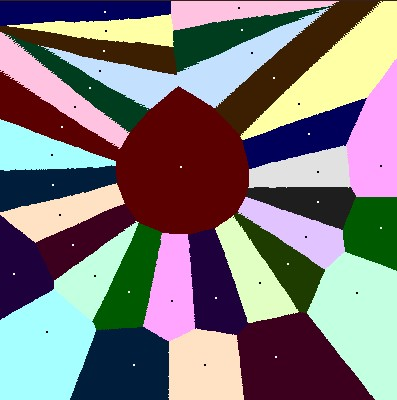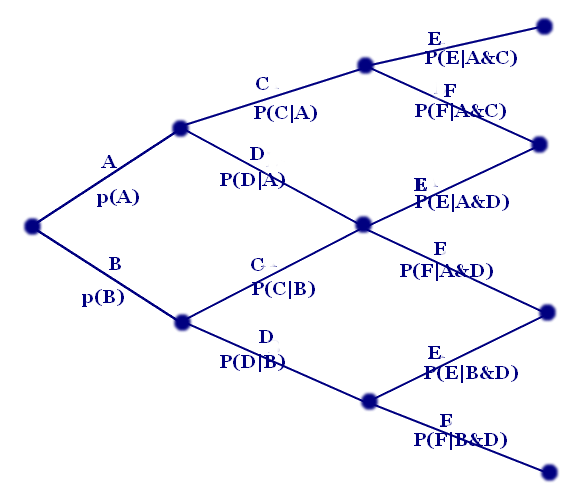# Probability: Multistep Experiments/ ...

Very often, the probabilities for a given event depend on the situation we are in. If we wake up and the sun is shining, our prediction of the temparature at noon will be different than if it is raining in the morning. This leads to the definition of a situation---the combination of all what is known so far--- and also of conditional probabilities. Given a situation S, and an experiment we perform in this situation with outcomes A and B, p(A|S) is the probability that outcome occurs, given the situation S, and p(B|S) is the probability of B in that situation.

### Probability TreesThis definition is usually applied in the situation of two or more experiments which are performed sequentially, so-called multistep experiments. Assume we first have an experiment with possible oucomes A and B. After then, a second experiment is performed with possible outcomes C and D. The graph to the right visualizes the situation, and it is called a probability tree. Starting with the leftmost vertex, one of the two vertices in the middle are reached after the first experiment, and then one of the four vertices to the right can be reached. The probabilities for the corresponding outcome are written below the corresponding line. Note again that the probabilities p(C|A) and p(C|B) do not have be identical, and the same for p(D|A) and p(D|B).

Totally, for the combined experiment, four different outcomes are possible: A and C (A&C), A and D (A&D), B and C (B&C), and B and D (B&D). How likely is each? It turns out that the total propability for each of the combined outcomes, of the four vertices to the right, are the products of the probabilities of the path leading to this vertex when starting at the leftmost vertex. Therefore

• p(A&C) = p(A)·p(C|A)
• p(A&D) = p(A)·p(D|A)
• p(B&C) = p(B)·p(C|B)
• p(B&C) = p(B)·p(D|B)

Aids Testing Example:..........
Example: A deck of 52 cards is available. The first experiments consists of drawing a card. The possible outcomes are red or black. Thus A is the outcome of having a red card drawn in the first drawing, and B is the outcome of this card being black. Then another card is drawn from the remaining 51 cards. C is the event that this second card is red, and D that this second card is black.
Obviously p(A)=p(B)=26/52. However p(C|A), the probability for a second red card if one red card has already been drawn, equals p(C|A)=25/51, but p(D|A)=26/51, p(C|B)=26/51, and p(D|B)=25/51. Therefore the combined event of two red cards A&C has the probability p(A&C) = p(A)·p(C|A) = 26/52·25/51. The same for the combined event B&D of two black cards; p(B&D) = p(B)·p(D|B) = 26/52·25/51. The event A&D means we draw a red card in the first round and a black card in the second round; it's probability equals p(A&D) = p(A)·p(D|A) = 26/52·26/51. The same probability we get for the event B&C for a black card in the first round and a red card in the second round. Therefore the probability for two different cards in both rounds equals p(A&D)+p(B&C) = 26/52·26/51+ 26/52·26/51 = 26/51, slightly higher than 1/2.

### Probability DigraphsIf there are many rounds of experiments, or many events in each experiment, then these game trees may become rather large. Let's say we draw three cards from a stack. A, C, respectively E mean a red card in the first, second, respectively third drawing, and B, D, respectively F mean a black card in the first, second, respectively third drawing. Assume that at the end we don't care about the ordering but rather only on how many black and red cards are drawn altogether. Therefore we don't distinguish between A&C&F and A&D&E and B&C&E---in each of these three cases we have two red cards and one black one. Also after the second drawing we don't have to distinguish between the situation A&D --- a red card in the first drawing and a black one in the second--- and B&C---a black card in the first drawing and a red one in the second. In both cases we have one red and one black cards in the hand, and 25 red cards and 25 black cards remaining in the stack. So the prospects for the future, for the third drawing are also identical. This is expressed by the fact that both subtrees starting at A&D respectively B&C are identical. In these cases we are allowed to identify these states (and also the subtrees starting at these vertices). What we get by doing so is called a probability digraph.Now in such a digraph, the probability for a combined event like A&C&F would be obtained like this. You look at all paths starting at the leftmost (start) vertex, always moving to the right, and arriving at the vertex in question. For each of these paths you multiply the probabilities along all edges. And then you make the sum of all these products, summed over vall these paths. In the example above, there are three such paths, A,C,F, A,D,E, and B,C,E. Therefore P(A&C&F=A&D&E=B&C&E) = p(A)·p(C|A)·p(F|A&C) + p(A)·p(D|A)·p(E|A&D) + p(B)·p(C|B)·p(E|B&C).

If you draw three cards from a stack of 52 cards, the probability of getting 2 red cards and 1 black one equals (1/2)·(25/51)·(26/50) + (1/2)·(26/51)·(25/50) + (1/2)·(26/51)·(25/50).

Please see the following for another application of this method, and also for remarks on the history of probability theory.

Another case where these probability trees or digraphs are applied is Decision Theory, which has a slightly different feature. Instead of having several rounds of random experiments, there are several alternating rounds with a human "player" making decisions, and with random experiments. Trees and digraphs are again used, but the analysis is slightly different.

### Independent or dependent events

Two events A and C of different experiments are called independent if p(A&C)=p(A)·p(C), or in other words, if p(C)=P(C|A).

• ...

### Exercises

1. In Franklin College, 40% of the freshmen are enrolled in a mathematics course, and 75% are enrolled in an English course, and 20% are taking both.
a) What is the probability that a randomly selected freshman is takijng an English course if it is known that he or she is enrolled in a mathematics course?
b) If a randomly selected freshman is taking an English course, what is the probability that he or she is also enrolled in a mathematics course?
c) Are the two events to be enrolled in an English course, and to be enrolled in a mathematics course independent events for freshman?
2. In which of the following cases are the events A and B independent? Give a short reason in each case.
a) A: student's car has a flat tire on her way to work, B: student is late to (:30 class.
b) p(A)=0.3, p(B)=3/10.
c) p(A)=0.5, p(not B)=0.7.
d) A: a student owns an automobile, B: a student is left-handed.
3. A random number generator produces a sequence of three digits, where each one of the digits 0, ... 9 has equal probability. Each digit is generated independently of the others.
Draw the probability tree for this three-step experiment.
Find the probability that a sequence
a) consists of all ones, or
b) consists of all odd integers.
4. Five number digits are generated in five rounds. In the first round, and digit from 0, ... 9 could be selected, with equal probability. In each further round, if a1...an has already been selected, then the next number selected could only be n+1, ... 9 with all these choices having equal probability. In other words, the digits of the number generated will always increase, like in 35689 or 24789.
Draw the probability tree for this three-step experiment.
Find the probability that a sequence
a) consists of all even integers, or
b) consists of all odd integers.
5. There are eight balls in an urn, identical except the color. Three of them are blue, three of them red, and two of them green. Consider the three-step experiment of
• drawing one ball, setting it aside,
• drawing another ball, and setting it aside, and
• drawing a third ball, and setting it aside.
a) Draw the probability tree for this three-step experiment.
b) How likely is it that two of the drawn balls are blue, and one of them is green?
c) How likely is it that all three balls have different colors.
d) How likely is it that all three balls have the same color.
6. a) Draw a probability tree for all the possible head-tail sequences that can occur when you flip a coin four times.
b) How many sequences contain exactly two heads?
c) How many sequences contain exactly three heads?
d) Draw the probability digraph for the case where you are only interested in how many heads a sequence occurs, not when they occur. That is, you would identify the situations HT and TH, you would identify HHT and HTH and THH, and so on.
e) What is the probability to get exactly two heads in a sequence of four attempts?